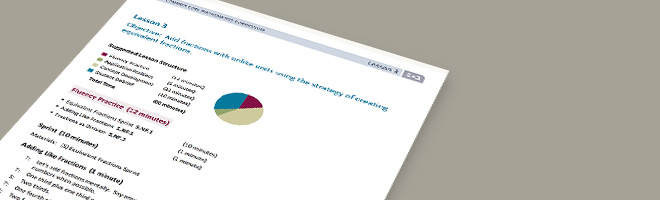• 01/02/14   |   Adjusted: 01/10/17   |   1 file# Add Fractions with Unlike Units Using the Strategy of Creating Equivalent Fractions

Author: Great Minds, as featured on EngageNY

• Description
• Files

Mathematically:

• Provides concrete examples for the need of like denominators for addition and subtraction of fractions
• Allows students to understand adding fractions with unlike denominators without going straight to the least common denominator
• Asks students to reason about how the size of units changes as they create equivalent fractions
• Encourages students to look for and make use of structure as they analyze rectangular fraction models (MP.7)

In the classroom:

• Uses concrete and pictorial models, particularly the rectangular fraction model, to make the mathematics of the lesson explicit
• Prompts students to share their developing thinking
• Allows for whole group, partner, and individual work in one lesson
• Gives formal and informal opportunities for teachers to check for understanding

• Making the Shifts

How does this lesson exemplify the instructional Shifts required by CCSSM?

 Focus Belongs to the major work of fifth grade Coherence Builds on key understandings of equivalent fractions (4.NF.A.1) and addition of fractions with like denominators (4.NF.B). Builds foundation toward adding and subtracting rational numbers (7.NS.A.1) Rigor Conceptual Understanding: primary in this lesson Procedural Skill and Fluency: secondary in this lesson Application: secondary in this lesson